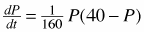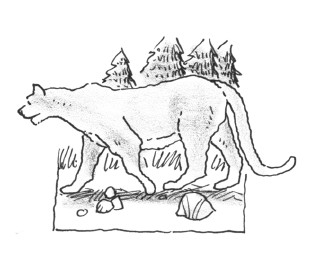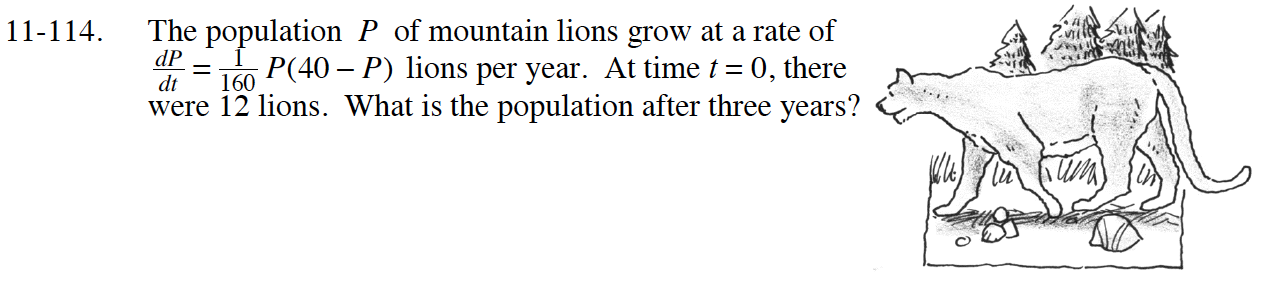### Home > CALC > Chapter 11 > Lesson 11.3.2 > Problem11-114

11-114.

The population P of mountain lions grow at a rate oflions per year. At time t = 0, there were 12 lions. What is the population after three years? Homework Help ✎Partial fraction decomposition needs to be used.

$160\int\Big(\frac{A}{P}+\frac{B}{40-P}\Big)dP=\int dt$

Solve for C when t = 0 and P = 12.

Substitute your value for C into the equation from Step 4 along with t = 3. Then solve for P.